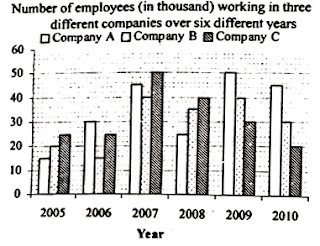### India Post, IBPS Clerk-Data Interpretation Quiz

Directions (Q. 01-05): Study the following graph carefully and answer the questions given below:1). The total number of employees in Company A over the years together was approximately what per cent of the total number of employees in Company C in the year 2007 and 2008 together?
a) 210%
b) 225%
c) 45%
d) 233%
e) 155%

2). What was the percentage decrease in the number of employees in Company B in the year 2008 in comparison to the previous year?
a) 22%
b) 10.5%
c) 12.5%
d) 25%
e) 27.5%

3). What was the ratio of the number of employees in Company B in the year 2005 to the number of the employees in Company C in the year 2007?
a) 5 : 4
b) 2:5
c) 4:5
d) 2:7
e) 3:5

4). If 30 per cent of the total number of employees in the year 2006 in all the companies together were females, what was the total number of male employees that year in all the companies together?
a) 4900
b) 59000
c) 48000
d) 49000
e) 48500

5). What is the ratio of the total employees in all three companies in 2007 to that in 2009?
a) 9 : 8
b) 7:6
c) 5:4
d) 6:5
e) 4:3

Directions Q 6- 10: The bar graph provided below gives the data of the production of paper (in lakh tonnes) by three different companies X, Y, Z over the years. Study the bar chart and answer the questions.6). What is the difference between the production of Company A in 2004 and Company B in 2003?
a) 10,00,000 tonnes
b) 15,00,000 tonnes
c) 20,00,000 tonnes
d) 25,00,000 tonnes
e) 5,00,000 tonnes

7). What is the ratio of the average production of Company C in the period 2002 - 2005 to the average production of company A in the same period?

a) 47:46
b) 49:47
c) 46:47
d) 49:46
e) 46:49

8). What is the percentage increase in the production of Company C from 2003 to 2005?
a) 27%
b) 26%
c) 28%
d) 25%
e) 30%

9). The average production for five years was maximum for which Company?
a) C
b) A
c) B
d) A and C
e) B and C

10). In which year the percentage of production of Company B to the production of company A is maximum?
a) 2004
b) 2002
c) 2001
d) 2005
e) 2003

Answer
1. D); Total number of employees in Company A over all the years together
=(15+30+45+25+50+45)x 1000 =210000
Total number of employees in Company C in the year 2007 and 2008
= (50 +40) x 1000=90000
Reqd % = (210000x100/90000)=233.3% =Approx. 233%

2. C); Number of employees in Company B in the year 2008=35000
Number of employees in Company B in the year 2007=40000
%decrease = (40 -35)/40 x (1000/1000) x 100=12.5%

3. B); Number of employees in Company B in the year 2005=20000
Number of employees in Company C in the year 2007=50000
:. Reqd ratio =20000 : 50000 =2 : 5

4. D); Total number of employees in 2006 in all the companies together
=(30 +15 +25)x1000=70000
Number of female employees = 70000 x (30/100) = 21000
:. Number of male employees = (70000 - 21000)
=49000

5. A); Total number of employees in Company A, B and C in 2007
=(45 + 40 + 50) x 1000 =135000
Total number of employees in Company A, B and C in 2009 = (50 + 40 + 30) x 1000
=120000
Reqd ratio = 135000 :120000 =9 : 8

6. b
As per bar chart, Difference will be
[80-65]*100000 = 15,00,000 tonnes
Please note: we multiplied it by 100000, because graph is given in "lakh" tones

7. d
Average production of Company A in the period 2002 – 2005 = ( 55+ 60+ 65+ 50)/4= 57.5
Average production of Company C in the period 2002 – 2005 = (45+ 55+ 75+ 70)/4 =61.25
Required ratio = 61.25 : 57.5 = 2.45 : 2.30 = 49: 46

8. a
Percentage increase in the production of Company C from 2003 to 2005 = ((70-55) / 55) * 100
=(15/55) *100 = 27.27 % ≈ 27%

9. c
Average production of Company A in the period 2001 – 2005 = (70+ 55+ 60+ 65+ 50)/5= 60
Average production of Company B in the period 2001 – 2005 = (55+ 65+ 80+45+ 65)/5= 62
Average production of Company C in the period 2001 – 2005 = (60+45+ 55+ 75+ 70)/5 =61
So company B has the maximum average.

10. e
2001 → % = (55 /70)*100= 78.5 %
2002 → % = (65 / 55)*100= 118.2 %
2003→ % = (80/60)*100= 133.3%
2004→ % = (45/65)*100 = 69.2%
2005→ % = (65/50)*100= 130%
So for 2003 the percentage is highest.